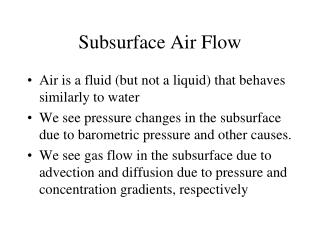DownloadDownload PresentationSubsurface Air Flow

# Subsurface Air Flow

Download Presentation## Subsurface Air Flow

- - - - - - - - - - - - - - - - - - - - - - - - - - - E N D - - - - - - - - - - - - - - - - - - - - - - - - - - -
##### Presentation Transcript

1. Subsurface Air Flow • Air is a fluid (but not a liquid) that behaves similarly to water • We see pressure changes in the subsurface due to barometric pressure and other causes. • We see gas flow in the subsurface due to advection and diffusion due to pressure and concentration gradients, respectively

2. Reasons for Studying Subsurface Airflow • Affects water levels in wells • Diffusion of radon gas into basements • Groundwater contamination by volatile gasses diffuses upward into homes • Subsurface contaminant remediation using forced air ventilation • Unsaturated zone testing

3. Darcy’s Law for Gasses • Replace the total head with pressure • p =  h •  = g is the manometer fluid specific weight •  is the density • g is gravity • Replace the hydraulic conductivity with the intrinsic permeability • K = k (/) •  is the fluid viscosity • Substitution yields: • q = - k/ p

4. Air Flow Parameters • Pressure, units of Pascals • 1 hPa = 1 mbar • 1 kPa = 1 cbar • 1 bar = 100 cbar = 1000 mbar • 1 atmosphere = 1.033 bars • Permeability, units of m2 • 1 m2 = 1 x 10-12 m2  1 darcy • Viscosity, units of Pa s • air  1.73 x 10-5 • water  1.0 x 10-3 • water is about 50 times more viscous than air • but it’s a function of temperature!!

5. Differences from Water • Not affected by gravity • We normally neglect the elevation head • Can make a difference for heavy gasses • Poiseuille’s Law is a little different • For water, the velocity is negligible next to a boundary • For air, the mean free path is so long, longer than the pore size, that the velocity next to the boundary is the same as in the middle. • This is called the Klinkenberg effect • The permeability for air is slightly larger than for water

6. Air Flow Equations • Steady flow conditions: • linear (z) • infinite cylinder (r) • finite cylinder (r, z) • spheroidal {oblate, prolate} (r1, r2) • Unsteady flow conditions: • pneumatic diffusivity, D = k / C • air compressibility, C • ideal gas law • isothermal conditions

7. Ideal Gas Law • P V = n R T • P is gas pressure • V is gas volume • n is number of moles • R is universal gas constant • T is temperature • P = (n / V) R T =  R T •  = n / V is the gas density, moles per volume • For two locations: • P1 = 1 R T1 and P2 = 2 R T2

8. Isothermal vs. Adiabatic • If the system is isothermal, T1 = T2 • P1 / P2 = 1 / 2 • which means that we can simplify! • If the system is adiabatic, T1 T2 • P1 / P2 = (1 / 2) n • appropriate for conditions of constant energy

9. Gas Transport • Forced Advection: • a pressure gradient pushes/pulls the gas • Free Advection: • a density gradient causes gas to sink or fall. • Dispersion: • a pressure gradient causes the spreading of the gas • Diffusion: • a concentration gradient causes the spreading of the gas

10. Question: Does humid air rise or sink in dry air? • Hint: Water vapor is less dense than dry air • If the two air masses are initially at the same pressure, then the wet air is less dense than the dry air, so it rises. • If the two dry air masses initially occupy equivalent volumes, and the water is then added to one air mass (say be evaporation) but not the other, then the pressure goes up, so that the wet air is at a higher pressure than the dry air, so the wet air pushes the dry air upward.

11. Gas Diffusion • Heavier gasses diffuse more slowly than lighter gasses • Helium has an atomic weight of 4 g/mole • Air is about 29 g/mole • Argon is 40 g/mole • Krypton is 83.8 g/mole • SF6 is 146 g/mole • Radon is 222 g/mole

12. In Heterogeneous Media • Heavier molecules • will advect more than they will diffuse • they will stay in the macropores • Lighter molecules • will diffuse more than they will advect • they will stay in the micropores • A function of the Peclet Number: • Pe = v L / D